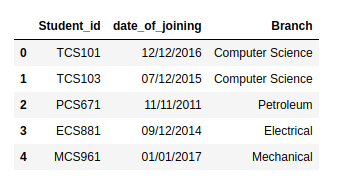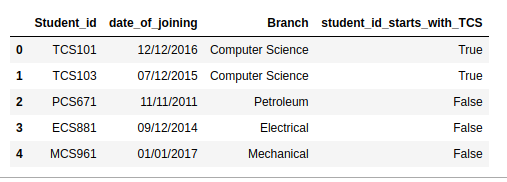# Check if a column starts with given string in Pandas DataFrame?

• Last Updated : 01 Aug, 2020

In this program, we are trying to check whether the specified column in the given data frame starts with specified string or not. Let us try to understand this using an example suppose we have a dataset named student_id, date_of_joining, branch.
Example:

## Python3

 `#importing library pandas as pd``import` `pandas as pd`` ` ` ` `#creating data frame for student``df ``=` `pd.DataFrame({``    ``'Student_id'``: [``'TCS101'``,``'TCS103'``, ``'PCS671'``, ``                   ``'ECS881'``, ``'MCS961'``],``     ` `    ``'date_of_joining'``: [``'12/12/2016'``,``'07/12/2015'``,``                        ``'11/11/2011'``,``'09/12/2014'``,``                        ``'01/01/2017'``],``     ` `    ``'Branch'``: [``'Computer Science'``,``'Computer Science'``,``               ``'Petroleum'``,``'Electrical'``,``'Mechanical'``]``})`` ` `# printing the given data frame``df`

Output:Now we want to know whether student_id starts with  TCS or not. Now let us try to implement this using Python

## Python3

 `#importing library pandas as pd``import` `pandas as pd`` ` ` ` `#creating data frame for student``df ``=` `pd.DataFrame({``    ``'Student_id'``: [``'TCS101'``,``'TCS103'``, ``'PCS671'``, ``                   ``'ECS881'``, ``'MCS961'``],``     ` `    ``'date_of_joining'``: [``'12/12/2016'``,``'07/12/2015'``,``                        ``'11/11/2011'``,``'09/12/2014'``,``                        ``'01/01/2017'``],``     ` `    ``'Branch'``: [``'Computer Science'``,``'Computer Science'``,``               ``'Petroleum'``,``'Electrical'``,``'Mechanical'``]``})`` ` `# joining new column in dataframe ``# .startswith function used to check``df[``'student_id_starts_with_TCS'``] ``=` `list``(``    ``map``(``lambda` `x: x.startswith(``'TCS'``), df[``'Student_id'``])) `` ` `# printing new data frame``df`

Output:In the above code, we used .startswith() function to check whether the values in the column starts with the given string. The .startswith() method in Python returns True if the string starts with the specified value, if not it returns False.

My Personal Notes arrow_drop_up